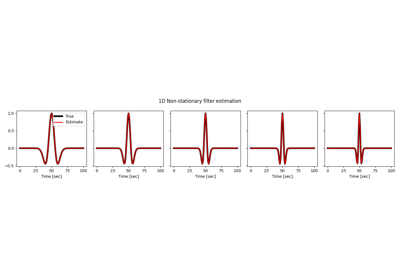# pylops.signalprocessing.NonStationaryFilters2D#

class pylops.signalprocessing.NonStationaryFilters2D(inp, hshape, ihx, ihz, engine='numpy', num_threads_per_blocks=(32, 32), dtype='float64', name='C')[source]#

2D non-stationary filter estimation operator.

Estimate a non-stationary two-dimensional filter by non-stationary convolution.

Parameters
inpnumpy.ndarray

Fixed input signal of size $$n_x \times n_z$$.

hshapelist or tuple

Shape of the 2d compact filters (filters must have odd number of samples and are assumed to be centered in the middle of the filter support).

ihxtuple

Indices of the x locations of the filters hs in the model (and data). Note that the filters must be regularly sampled, i.e. $$dh_x=\text{diff}(ihx)=\text{const.}$$

ihztuple

Indices of the z locations of the filters hs in the model (and data). Note that the filters must be regularly sampled, i.e. $$dh_z=\text{diff}(ihz)=\text{const.}$$

enginestr, optional

Engine used for spread computation (numpy, numba, or cuda)

num_threads_per_blockstuple, optional

Number of threads in each block (only when engine=cuda)

dtypestr, optional

Type of elements in input array.

namestr, optional

Name of operator (to be used by pylops.utils.describe.describe)

Raises
ValueError

If filters hs have even size

ValueError

If ihx or ihz is not regularly sampled

NotImplementedError

If engine is neither numpy, fftw, nor scipy.

Notes

The NonStationaryConvolve2D operator is used to estimate a non-stationary two-dimensional filter betwen two signals, an input signal (provided directly to the operator) and the desired output signal.

For more details on the numerical implementation of the forward and adjoint, see pylops.signalprocessing.NonStationaryFilters1D.

Attributes
shapetuple

Operator shape

explicitbool

Operator contains a matrix that can be solved explicitly (True) or not (False)

Methods

 __init__(inp, hshape, ihx, ihz[, engine, ...]) adjoint() apply_columns(cols) Apply subset of columns of operator cond([uselobpcg]) Condition number of linear operator. conj() Complex conjugate operator div(y[, niter, densesolver]) Solve the linear problem $$\mathbf{y}=\mathbf{A}\mathbf{x}$$. dot(x) Matrix-matrix or matrix-vector multiplication. eigs([neigs, symmetric, niter, uselobpcg]) Most significant eigenvalues of linear operator. matmat(X) Matrix-matrix multiplication. matvec(x) Matrix-vector multiplication. reset_count() Reset counters rmatmat(X) Matrix-matrix multiplication. rmatvec(x) Adjoint matrix-vector multiplication. todense([backend]) Return dense matrix. toimag([forw, adj]) Imag operator toreal([forw, adj]) Real operator tosparse() Return sparse matrix. trace([neval, method, backend]) Trace of linear operator. transpose()

## Examples using pylops.signalprocessing.NonStationaryFilters2D#Non-stationary Filter Estimation

Non-stationary Filter Estimation